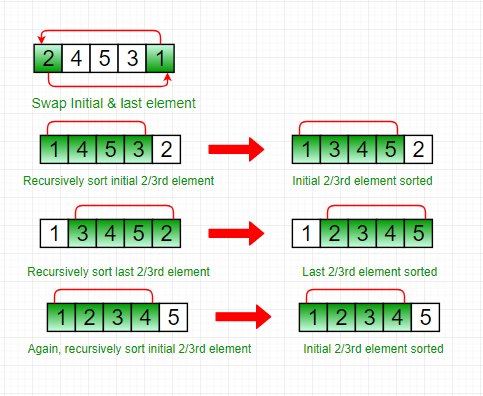Related Articles
Stooge Sort
• Difficulty Level : Hard
• Last Updated : 04 Apr, 2018

The Stooge sort is a recursive sorting algorithm. It is defined as below (for ascending order sorting).

```Step 1 : If value at index 0 is greater than
value at last index, swap them.
Step 2:  Recursively,
a) Stooge sort the initial 2/3rd of the array.
b) Stooge sort the last 2/3rd of the array.
c) Stooge sort the initial 2/3rd again to confirm.
```

NOTE: Always take the ceil of ((2/3)*N) for selecting elements.

## Recommended: Please try your approach on {IDE} first, before moving on to the solution.

Illustration:

```Input :   2 4 5 3 1
Output : 1 2 3 4 5
Explanation:
Initially, swap 2 and 1 following above step 1.
1 4 5 3 2
Now, recursively sort initial 2/3rd of the elements.
1 4 5 3 2
1 3 4 5 2
Then, recursively sort last 2/3rd of the elements.
1 3 4 5 2
1 2 3 4 5
Again, sort the initial 2/3rd of the elements to confirm final data is sorted.
1 2 3 4 5```## C++

 `// C++ code to implement stooge sort``#include ``using` `namespace` `std;`` ` `// Function to implement stooge sort``void` `stoogesort(``int` `arr[], ``int` `l, ``int` `h)``{``    ``if` `(l >= h)``        ``return``;`` ` `    ``// If first element is smaller than last,``    ``// swap them``    ``if` `(arr[l] > arr[h])``        ``swap(arr[l], arr[h]);`` ` `    ``// If there are more than 2 elements in``    ``// the array``    ``if` `(h - l + 1 > 2) {``        ``int` `t = (h - l + 1) / 3;`` ` `        ``// Recursively sort first 2/3 elements``        ``stoogesort(arr, l, h - t);`` ` `        ``// Recursively sort last 2/3 elements``        ``stoogesort(arr, l + t, h);`` ` `        ``// Recursively sort first 2/3 elements``        ``// again to confirm``        ``stoogesort(arr, l, h - t);``    ``}``}`` ` `// Driver Code``int` `main()``{``    ``int` `arr[] = { 2, 4, 5, 3, 1 };``    ``int` `n = ``sizeof``(arr) / ``sizeof``(arr);`` ` `    ``// Calling Stooge Sort function to sort``    ``// the array``    ``stoogesort(arr, 0, n - 1);`` ` `    ``// Display the sorted array``    ``for` `(``int` `i = 0; i < n; i++)``        ``cout << arr[i] << ``" "``;`` ` `    ``return` `0;``}`

## Java

 `// Java program to implement stooge sort``import` `java.io.*;`` ` `public` `class` `stooge {``    ``// Function to implement stooge sort``    ``static` `void` `stoogesort(``int` `arr[], ``int` `l, ``int` `h)``    ``{``        ``if` `(l >= h)``            ``return``;`` ` `        ``// If first element is smaller``        ``// than last, swap them``        ``if` `(arr[l] > arr[h]) {``            ``int` `t = arr[l];``            ``arr[l] = arr[h];``            ``arr[h] = t;``        ``}`` ` `        ``// If there are more than 2 elements in``        ``// the array``        ``if` `(h - l + ``1` `> ``2``) {``            ``int` `t = (h - l + ``1``) / ``3``;`` ` `            ``// Recursively sort first 2/3 elements``            ``stoogesort(arr, l, h - t);`` ` `            ``// Recursively sort last 2/3 elements``            ``stoogesort(arr, l + t, h);`` ` `            ``// Recursively sort first 2/3 elements``            ``// again to confirm``            ``stoogesort(arr, l, h - t);``        ``}``    ``}`` ` `    ``// Driver Code``    ``public` `static` `void` `main(String args[])``    ``{``        ``int` `arr[] = { ``2``, ``4``, ``5``, ``3``, ``1` `};``        ``int` `n = arr.length;`` ` `        ``stoogesort(arr, ``0``, n - ``1``);`` ` `        ``for` `(``int` `i = ``0``; i < n; i++)``            ``System.out.print(arr[i] + ``" "``);``    ``}``}``// Code Contributed by Mohit Gupta_OMG <(0_o)>`

## Python3

 `# Python program to implement stooge sort`` ` `def` `stoogesort(arr, l, h):``    ``if` `l >``=` `h:``        ``return``  ` `    ``# If first element is smaller``    ``# than last, swap them``    ``if` `arr[l]>arr[h]:``        ``t ``=` `arr[l]``        ``arr[l] ``=` `arr[h]``        ``arr[h] ``=` `t``  ` `    ``# If there are more than 2 elements in``    ``# the array``    ``if` `h``-``l ``+` `1` `> ``2``:``        ``t ``=` `(``int``)((h``-``l ``+` `1``)``/``3``)``  ` `        ``# Recursively sort first 2 / 3 elements``        ``stoogesort(arr, l, (h``-``t))``  ` `        ``# Recursively sort last 2 / 3 elements``        ``stoogesort(arr, l ``+` `t, (h))``  ` `        ``# Recursively sort first 2 / 3 elements``        ``# again to confirm``        ``stoogesort(arr, l, (h``-``t))``  ` ` ` `# deriver ``arr ``=` `[``2``, ``4``, ``5``, ``3``, ``1``]``n ``=` `len``(arr)`` ` `stoogesort(arr, ``0``, n``-``1``)``  ` `for` `i ``in` `range``(``0``, n):``    ``print``(arr[i], end ``=` `' '``)`` ` `# Code Contributed by Mohit Gupta_OMG <(0_o)>`

## C#

 `// C# program to implement stooge sort``using` `System;`` ` `class` `GFG {``     ` `    ``// Function to implement stooge sort``    ``static` `void` `stoogesort(``int``[] arr,``                            ``int` `l, ``int` `h)``    ``{``        ``if` `(l >= h)``            ``return``;`` ` `        ``// If first element is smaller``        ``// than last, swap them``        ``if` `(arr[l] > arr[h]) {``            ``int` `t = arr[l];``            ``arr[l] = arr[h];``            ``arr[h] = t;``        ``}`` ` `        ``// If there are more than 2 ``        ``// elements in the array``        ``if` `(h - l + 1 > 2) {``            ``int` `t = (h - l + 1) / 3;`` ` `            ``// Recursively sort first ``            ``// 2/3 elements``            ``stoogesort(arr, l, h - t);`` ` `            ``// Recursively sort last``            ``// 2/3 elements``            ``stoogesort(arr, l + t, h);`` ` `            ``// Recursively sort first ``            ``// 2/3 elements again to ``            ``// confirm``            ``stoogesort(arr, l, h - t);``        ``}``    ``}`` ` `    ``// Driver Code``    ``public` `static` `void` `Main()``    ``{``        ``int``[] arr = { 2, 4, 5, 3, 1 };``        ``int` `n = arr.Length;`` ` `        ``// Calling Stooge Sort function``        ``// to sort the array``        ``stoogesort(arr, 0, n - 1);`` ` `        ``// Display the sorted array``        ``for` `(``int` `i = 0; i < n; i++)``            ``Console.Write(arr[i] + ``" "``);``    ``}``}`` ` `// This code is contributed by Sam007.`

Output:

```1 2 3 4 5
```

The running time complexity of stooge sort can be written as,

T(n) = 3T(3n/2) + ?(1)

Solution of above recurrence is O(n(log3/log1.5)) = O(n2.709), hence it is slower than even bubble sort(n^2).

Reference:
Wikipedia

This article is contributed by DANISH KALEEM. If you like GeeksforGeeks and would like to contribute, you can also write an article using contribute.geeksforgeeks.org or mail your article to contribute@geeksforgeeks.org. See your article appearing on the GeeksforGeeks main page and help other Geeks.